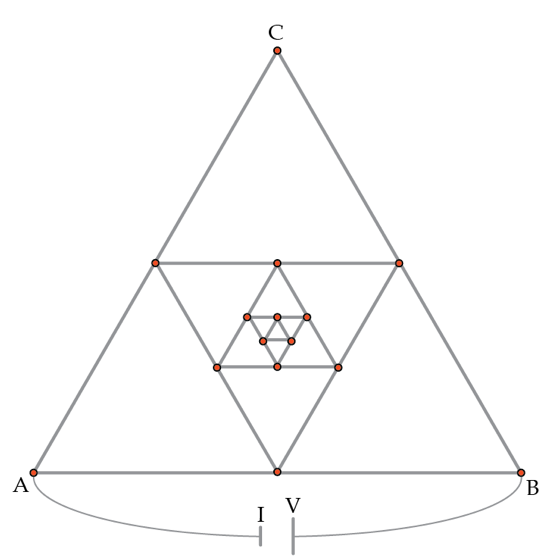# Power dissipated in a fractal circuit

Suppose we take three homogeneous wires with resistance $R=1~\Omega$ and build an equilateral triangle ABC. Then we take more wires and we inscribe a smaller equilateral triangle inside the original one and we repeat this procedure many times to obtain a fractal circuit as shown in the figure below. What will be the total power dissipated in the circuit in Watts if a $1~\mbox{V}$ voltage source (or negligible internal resistance) is connected across points A and B?×

Problem Loading...

Note Loading...

Set Loading...